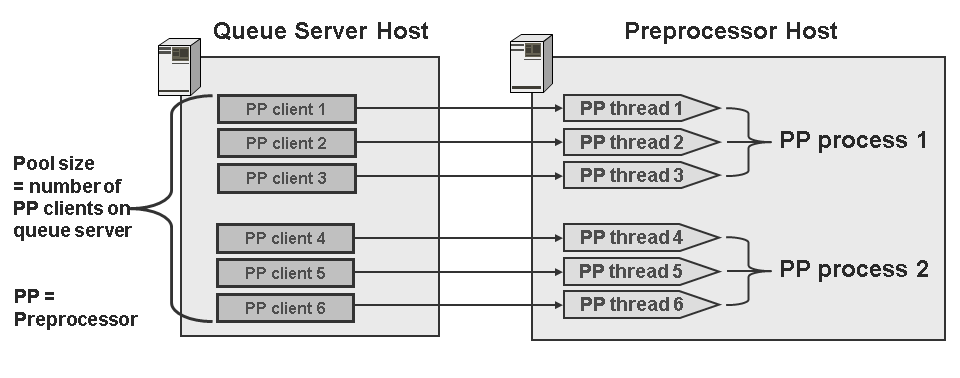Show TOC

# Preprocessor Threads and Queue Server Pool SizeUse

The pool size is important for achieving optimum integration between the queue servers and preprocessors. The pool size determines how many documents a queue server can distribute to the preprocessors at once.

From a technical point of view, the pool size determines how many preprocessor clients a queue server instantiates at startup. The preprocessor client is an internal component of the queue server. The queue server uses the preprocessor clients to communicate with the preprocessors and uses its services.

Depending on the number of preprocessor clients started in the queue server (= pool size), the corresponding number of preprocessor threads are started by a central worker thread management. You can use the pool size in the queue server to control the number of preprocessor threads on the hosts that preprocessors are running on.

The following relationship applies:

<queue server pool size> = <number of preprocessor threads>

Example

For example, if you set the pool size in the queue server to the value 6, the corresponding number of preprocessor threads are started on the host that the preprocessor(s) are running on. If two preprocessor processes are running there, the threads are distributed between two preprocessor processes, which correspond to three threads per process.What Value Should the Queue Server Pool Size Have?

You must determine the optimum pool size and thus the number of preprocessor threads individually for your system. For each preprocessor process, a maximum of three preprocessor threads should be started using the entry for the pool size.

Thus, the following relationship applies:

<number of preprocessor threads per preprocessor process> = 3. Since a maximum of only one preprocessor should be started for each CPU (see Number of Preprocessors and Preprocessor Threads), this results in the following relationship for a distributed system landscape with multiple queue servers and preprocessor hosts:

<total pool size of all queue servers>=

<total number of CPUs of all preprocessor hosts> * 3

If the pool size is too low, the preprocessor can have unnecessary idle times and not have a full load, although resources are still available. If the pool size is too large, the host on which the queue server is running uses too many system resources to manage the pool.

You should check the CPU load for the preprocessors for a while. If system resources are still available, you can increase the pool size to improve performance. However, if you increase the pool size beyond the recommendations, you gain no performance benefits and might actually cause performance to drop.

The pool size of queue servers is configured in the TREXQueueServer.ini file.